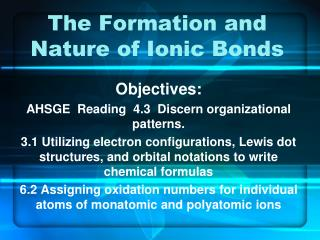DownloadDownload PresentationThe Formation and Nature of Ionic Bonds

The Formation and Nature of Ionic Bonds

Télécharger la présentationThe Formation and Nature of Ionic Bonds

- - - - - - - - - - - - - - - - - - - - - - - - - - - E N D - - - - - - - - - - - - - - - - - - - - - - - - - - -
Presentation Transcript

1. The Formation and Nature of Ionic Bonds Objectives: AHSGE Reading 4.3 Discern organizational patterns. 3.1 Utilizing electron configurations, Lewis dot structures, and orbital notations to write chemical formulas 6.2 Assigning oxidation numbers for individual atoms of monatomic and polyatomic ions

2. Formation of an Ionic Bond • Define “ionic bond”. • Answer: the electrostatic force that holds oppositely charged particles together in an ionic compound • Binary ionic compounds contain ____ different elements. • MgO? • CaSO4? • In ionic compounds, one “part” must gain electrons, and one “part” must lose electrons. WHY?????

3. Example: Ca + F • Which element will GAIN electrons to form an ion? • How many will it gain? • Write the formula for the ion formation? • What is the name of the ion? • How many will the other element lose when it forms an ion? • Write the formula for the ion formation. • What is the name of the ion?

4. Example: Ca + F (continued) • We determined that calcium loses 2 electrons, and fluorine gains 1. • If a fluoride ion can only take 1 of calcium’s electrons, what will calcium do with the other electron it loses? Answer: It will look for another ion to take it. • So how many fluoride ions does each calcium ion “want to” bond with? Answer: 2

5. Example: Ca + F (continued) • Mathematically : 1 Ca ion (+2) + 2 F ion (-1) = 1(+2) + 2(-1) = 0 Ca ion F ion • Find the least common multiple of the 2 oxidation numbers – in this case, 2. • The charges must balance so the whole compound is neutral (total charge = 0)! • The formula will have 1 Ca and 2 F ions, so it will be CaF2.

6. Example: Ca + F (continued) • The formula obtained is called the formula unit: the simplest ratio of the ions in an ionic compound. • Its name will be metal + nonmetal-ide, or calcium fluoride.

7. Formation of an Ionic Bond • 3 ways to show the formation of an ionic compound: • Electron configuration • Orbital notation • Electron-dot structure • Look at the opening review for this chapter!!! • [Ne] 3s1 + [Ne] 3s23p5 → [Ne] + [Ar] + nrg • What happens to the [Ne] 3s1? • What happens to the [Ne] 3s23p5? • Why is there energy released?

8. Formation of an Ionic Bond • Orbital notation • Write the orbital notations for both Na and Cl. • What do you think will happen to the extra electron in the 3s orbital of Na? • What will the new orbital notations look like? • Electron-dot structure • Write the electron-dot structures for both Na and Cl. • Write the electron-dot structures of the ions formed.

9. Practice Problems Assignment • Use the example from your notes (also found on p.216) and Example Problem on p.217 to complete Practice Problems 7-11, p.217. • You may use whichever method works best for you!!! • Complete #7 and let me check your work BEFORE YOU CONTINUE!

10. Nature of Ionic Bonds • Liquid forms are electrolytes: conduct an electrical current. (Why aren’t solids?) • Formation of ionic bonds is exothermic: ? • because the ionic compound formed is more stable and lower in energy than the individual ions • Hard, rigid, brittle solids with high melting points and boiling points because it requires a lot of energy to break those strong ionic bonds. • Electronegativity difference is > 1.6

11. Workbook Assignment • Pp. 44-45: OMIT 7-9, 19-23